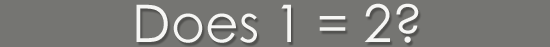Does 1 = 2?

1st Proof

 x = 1
set x equal to 1
 x2 = x
multiply both sides by x
 x2 - 1 = x - 1
subtract 1 from both sides
 (x - 1)(x + 1) = x - 1
separate left side into factors
 x + 1 = 1
divide both sides by (x - 1)
 1 + 1 = 1
substitute 1 for x
 2 = 1

2nd Proof

 (-1)(-1) = 1 the square of -1 is 1 -1 = 1/-1 divide both sides by -1 -1/1 = 1/-1 identity operation; for all real (or complex) x, x = x/1 i/1 = 1/i take the square root of both sides (i=sqrt(-1)) i/2 = 1/2i divide both sides by two i2/2 = i/2i multiply both sides by i -1/2 = 1/2 substitute -1 for i2 and 1 for i/i -1/2 + 3/2 = 1/2 + 3/2 add 1 1/2 (3/2) to both sides 1 = 2

3rd Proof

 x2 = x+x+x+...+x (x times) definition of x2; x not equal to zero 2x = 1+1+1+...+1 (x times) take derivative of both sides;  derivative of xn = nxn-1 2x = x x = 1+1+1+...+1 (x times) 2 = 1 divide both sides by x (x not equal to zero)